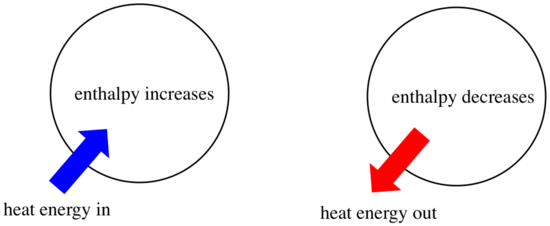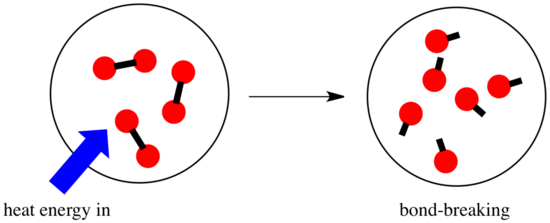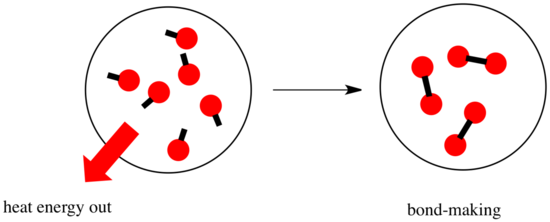# Enthalpy Changes in Reactions

$$\newcommand{\vecs}{\overset { \rightharpoonup} {\mathbf{#1}} }$$ $$\newcommand{\vecd}{\overset{-\!-\!\rightharpoonup}{\vphantom{a}\smash {#1}}}$$$$\newcommand{\id}{\mathrm{id}}$$ $$\newcommand{\Span}{\mathrm{span}}$$ $$\newcommand{\kernel}{\mathrm{null}\,}$$ $$\newcommand{\range}{\mathrm{range}\,}$$ $$\newcommand{\RealPart}{\mathrm{Re}}$$ $$\newcommand{\ImaginaryPart}{\mathrm{Im}}$$ $$\newcommand{\Argument}{\mathrm{Arg}}$$ $$\newcommand{\norm}{\| #1 \|}$$ $$\newcommand{\inner}{\langle #1, #2 \rangle}$$ $$\newcommand{\Span}{\mathrm{span}}$$ $$\newcommand{\id}{\mathrm{id}}$$ $$\newcommand{\Span}{\mathrm{span}}$$ $$\newcommand{\kernel}{\mathrm{null}\,}$$ $$\newcommand{\range}{\mathrm{range}\,}$$ $$\newcommand{\RealPart}{\mathrm{Re}}$$ $$\newcommand{\ImaginaryPart}{\mathrm{Im}}$$ $$\newcommand{\Argument}{\mathrm{Arg}}$$ $$\newcommand{\norm}{\| #1 \|}$$ $$\newcommand{\inner}{\langle #1, #2 \rangle}$$ $$\newcommand{\Span}{\mathrm{span}}$$$$\newcommand{\AA}{\unicode[.8,0]{x212B}}$$

Thermodynamics is the study of the relationship between heat (or energy) and work. Enthalpy is a central factor in thermodynamics. It is the heat content of a system. The heat that passes into or out of the system during a reaction is the enthalpy change. Whether the enthalpy of the system increases (i.e. when energy is added) or decreases (because energy is given off) is a crucial factor that determines whether a reaction can happen.Sometimes, we call the energy of the molecules undergoing change the "internal enthalpy". Sometimes, we call it the "enthalpy of the system." These two phrases refer to the same thing. Similarly, the energy of the molecules that do not take part in the reaction is called the "external enthalpy" or the "enthalpy of the surroundings".

Roughly speaking, the energy changes that we looked at in the introduction to thermodynamics were changes in enthalpy. We will see in the next section that there is another energetic factor, entropy, that we also need to consider in reactions. For now, we will just look at enthalpy.

• Enthalpy is the heat content of a system.
• The enthalpy change of a reaction is roughly equivalent to the amount of energy lost or gained during the reaction.
• A reaction is favored if the enthalpy of the system decreases over the reaction.

That last statement is a lot like the description of energetics on the previous page. If a system undergoes a reaction and gives off energy, its own energy content decreases. It has less energy left over if it gave some away. Why does the energy of a set of molecules change when a reaction occurs? To answer that, we need to think about what happens in a chemical reaction.

In a reaction, there is a change in chemical bonding. Some of the bonds in the reactants are broken, and new bonds are made to form the products. It costs energy to break bonds, but energy is released when new bonds are made.Whether a reaction is able to go forward may depend on the balance between these bond-making and bond-breaking steps.• A reaction is exothermic if more energy is released by formation of new bonds than is consumed by breaking old bonds.
• A reaction is exothermic if weaker bonds are traded for stronger ones.
• A reaction is endothermic if bond-breaking costs more energy than what is provided in bond-making.Bond energies (the amount of energy that must be added in order to break a bond) are an important factor in determining whether a reaction will occur. Bond strengths are not always easy to predict, because the strength of a bond depends on a number of factors. However, lots of people have done lots of work measuring bond strengths, and they have collected the information in tables, so if you need to know how strong a bond is, you can just look up the information you need.

Bond Bond Energy (kcal/mol) Bond Bond Energy (kcal/mol)
H-H 104 O-H 111
C-C 83 C-H 99
O=O 119 N-H 93
N=N 226 C=O 180

For example, suppose you wanted to know whether the combustion of methane were an exothermic or endothermic reaction. I am going to guess that it's exothermic, because this reaction (and others like it) is used to provide heat for lots of homes by burning natural gas in furnaces.

The "combustion" of methane means that it is burned in air, so that it reacts with oxygen. The products of burning hydrocarbons are mostly carbon dioxide and water. The carbon atom in methane (CH4) gets incorporated into a carbon dioxide molecule. The hydrogen atoms get incorporated into water molecules. There are four hydrogen atoms in methane, so that's enough to make two molecules of H2O.

• Four C-H bonds must be broken in the combustion of methane.
• Four new O-H bonds are made when the hydrogens from methane are added into new water molecules.
• Two new C=O bonds are made when the carbon from methane is added into a CO2 molecule.

The other piece of the puzzle is the oxygen source for the reaction. Oxygen is present in the atmosphere mostly as O2. Because we need two oxygen atoms in the CO2 molecule and two more oxygen atoms for the two water molecules, we need a total of four oxygen atoms for the reaction, which could be provided by two O2 molecules.

• Two O=O bonds must be broken to provide the oxygen atoms for the products.

Altogether, that's four C-H and two O=O bonds broken, plus two C=O and four O-H bonds made. That's 4 x 99 kcal/mol for the C-H bonds and 2 x 119 kcal/mol for the O=O bonds, a total of 634 kJ/mol added. The reaction releases 2 x 180 kcal/mol for the C=O bonds and 4 x 111 kcla/mol for the OH bonds, totaling 804 kcal/mol. Overall, there is 170 kcal/mol more released than is consumed.

That means the reaction is exothermic, so it produces heat. It's probably a good way to heat your home.

## Problem TD2.1.

Compare the combustion of ethane to the combustion of methane.

1. Write a reaction for the combustion of ethane, CH3CH3, to carbon dioxide and water.
2. How many carbon dioxide molecules would be produced from one molecule of ethane?
3. How many water molecules would be produced from one molecule of ethane?
4. How many oxygen molecules would be needed to provide oxygen atoms to accomplish the steps in questions (b) and (c)?
5. How much energy is consumed / produced by the reaction? Compare this result to the one for methane.

## Problem TD2.2.

The Haber-Bosch process is used to make ammonia for fertilizer. It employs the reaction of hydrogen gas (H2) with atmospheric nitrogen (N2) in a 3:1 ratio to produce ammonia (NH3).

1. Write a reaction for the Haber-Bosch process.
2. How many ammonia molecules would be produced from one molecule of nitrogen?
3. How much energy is consumed / produced by the reaction?